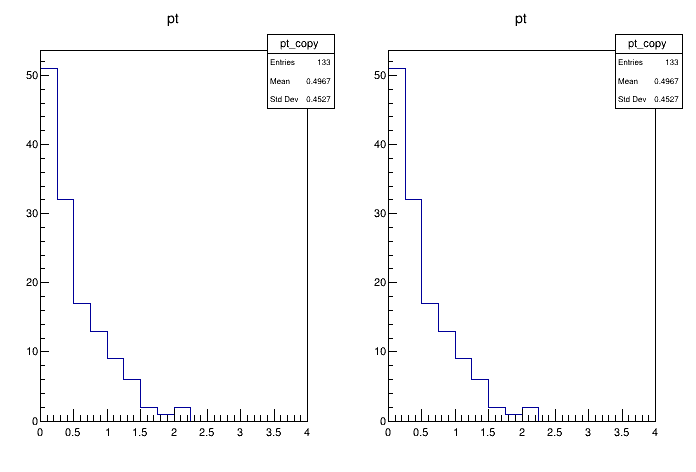# Df 0 1 7_Vec Ops H E P¶

This tutorial shows how VecOps can be used to slim down the programming model typically adopted in HEP for analysis.

Author: Danilo Piparo, Andre Vieira Silva
This notebook tutorial was automatically generated with ROOTBOOK-izer from the macro found in the ROOT repository on Monday, July 06, 2020 at 11:36 AM.

In :
import ROOT

filename = ROOT.gROOT.GetTutorialDir().Data() + "/dataframe/df017_vecOpsHEP.root"
treename = "myDataset"

def WithPyROOT(filename):
from math import sqrt
f = ROOT.TFile(filename)
h = ROOT.TH1F("pt", "pt", 16, 0, 4)
for event in f.myDataset:
for E, px, py in zip(event.E, event.px, event.py):
if (E > 100):
h.Fill(sqrt(px*px + py*py))
h.DrawCopy()

def WithRDataFrameVecOpsJit(treename, filename):
f = ROOT.RDataFrame(treename, filename)
h = f.Define("good_pt", "sqrt(px*px + py*py)[E>100]")\
.Histo1D(("pt", "pt", 16, 0, 4), "good_pt")
h.DrawCopy()

Welcome to JupyROOT 6.23/01


We plot twice the same quantity, the key is to look into the implementation of the functions above

In :
c = ROOT.TCanvas()
c.Divide(2,1)
c.cd(1)
WithPyROOT(filename)
c.cd(2)
WithRDataFrameVecOpsJit(treename, filename)


Draw all canvases

In :
from ROOT import gROOT
gROOT.GetListOfCanvases().Draw()